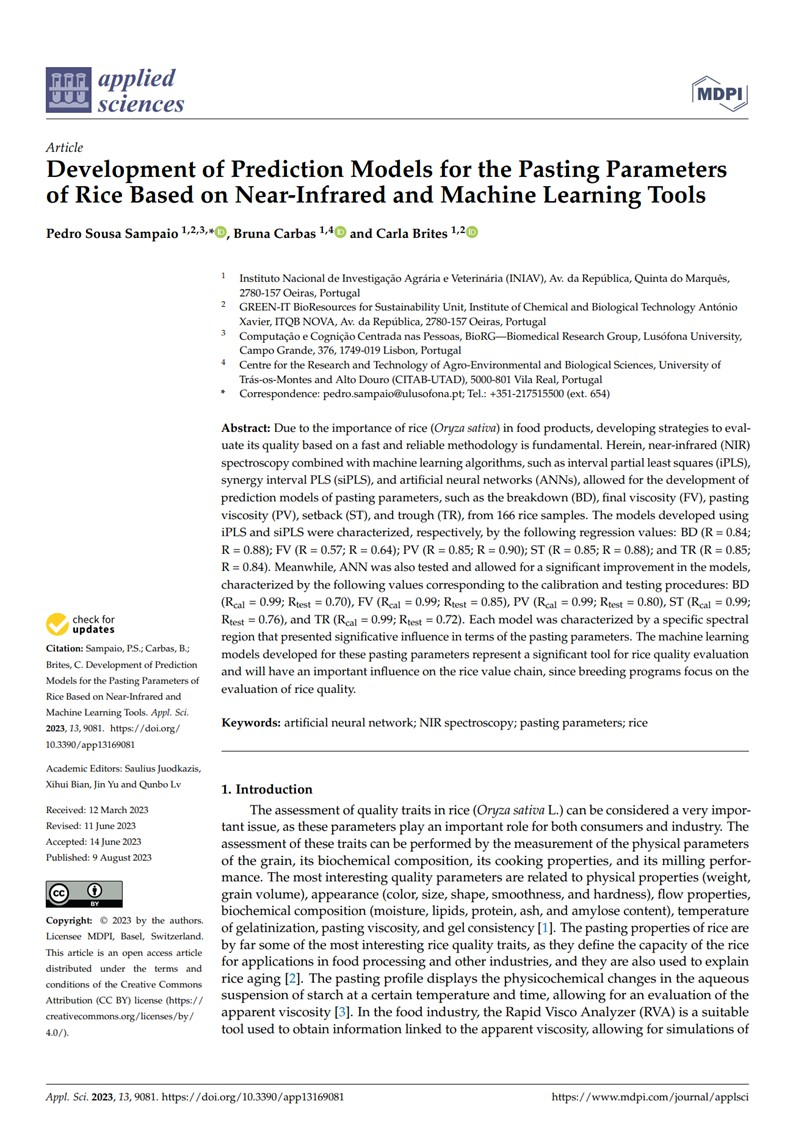# New article on prediction models

Due to the importance of rice (Oryza sativa) in food products, developing strategies to evaluate its quality based on a fast and reliable methodology is fundamental. Herein, near-infrared (NIR) spectroscopy combined with machine learning algorithms, such as interval partial least squares (iPLS), synergy interval PLS (siPLS), and artificial neural networks (ANNs), allowed for the development of prediction models of pasting parameters, such as the breakdown (BD), final viscosity (FV), pasting viscosity (PV), setback (ST), and trough (TR), from 166 rice samples. The models developed using iPLS and siPLS were characterized, respectively, by the following regression values: BD (R = 0.84; R = 0.88); FV (R = 0.57; R = 0.64); PV (R = 0.85; R = 0.90); ST (R = 0.85; R = 0.88); and TR (R = 0.85; R = 0.84). Meanwhile, ANN was also tested and allowed for a significant improvement in the models, characterized by the following values corresponding to the calibration and testing procedures: BD (Rcal = 0.99; Rtest = 0.70), FV (Rcal = 0.99; Rtest = 0.85), PV (Rcal = 0.99; Rtest = 0.80), ST (Rcal = 0.99; Rtest = 0.76), and TR (Rcal = 0.99; Rtest = 0.72). Each model was characterized by a specific spectral region that presented significative influence in terms of the pasting parameters. The machine learning models developed for these pasting parameters represent a significant tool for rice quality evaluation and will have an important influence on the rice value chain, since breeding programs focus on the evaluation of rice quality.